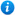* Watermarks do not appear on purchased resources

Preview resource: Click slides to enlarge

# Multiply by Eight

KS2 Maths Teaching Resources: Multiply by Eight.

Year 3 maths programme of study - Number (multiplication and division):

• recall and use multiplication and division facts for the 3, 4 and 8 multiplication tables

In 'Multiply by Eight' pupils practise the x 8 multiplication table.

'Multiply by Eight' is an animated PowerPoint presentation which includes:

• interactive activities
• x 8 flash cards
• 2 worksheets

'Multiply by Eight' can be fully edited allowing teachers to adapt, alter and revise it as much or as little as needed.

To preview 'Multiply by Eight' please click on the PowerPoint images.

Our Price : £1.99 / 2 Credits